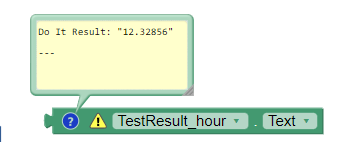# Fractional Hours into Hour and Minutes

Hi, I have a calculation that returns hour in fraction, like the one result shown in .png below, now I have to display final value in standard time format HH:MM:SS with the help of maketime block. My question is how can I take fractional value from the first calculation and add it into minutes, plus I may not want to round off the hours as it will result in wrong hour.

Why dont you use default block like this?

I need to calculate the Hours, Minutes and seconds. The problem is when I calculate hours it appears to be a fractional value and not a round number. I do not need to display system time.

Can you give any fractional value that need to convert into hhmmss?

Have you tried lik this?
How to Convert Decimal Hours to Time in HH:MM:SS

``````12.675 hours = 12 hours + .675 hours. full hours = 12.
``````

Then find the number of minutes.
minutes = .675 hours × 60 minutes. minutes = 40.5 minutes.
full minutes = 40. ...
seconds = .5 minutes × 60 seconds. seconds = 30 seconds.
Finally, rewrite as HH:MM:SS. time = 12:40:30.

Here you can see the hour value returned is 12.32856;This is exactly I want to achieve but how can I do using code blocks?

what about using some math blocks? you can find them in the Math drawer
Taifun

2 Likes
2 Likes

This topic was automatically closed 7 days after the last reply. New replies are no longer allowed.